# NCERT Solutions Class 4 Mathematics Chapter 9 Halves And Quarters

NCERT Solutions for Class 4 Mathematics Math Magic for Chapter 9 Halves And Quarters

1. If the cats ask you to divide the chapati equally how will you divide it?

Ans.To divide the chapatti equally, I will fold it in such a way that one part coincides with the other exactly. These parts will look as shown in the figure.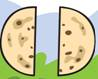2. If two or more cats come for food how will you divide one chapati equally for four cats?

Ans.To divide one chapati equally into four parts. I will first divide into two halves. Then. I will fold each half in such a way that one part coincides with the other exactly. These parts will look as under:3. Half of Many Pieces

Rani got a chocolate. She divided it equally and gave half to her friend Reena.

(a) Circle the portion the Reena got.

Ans. (a)Reena’s portion is shown as encircled: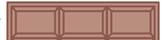(b) How many pieces of chocolate are there?

Ans. (b)There are six pieces of chocolate.

(c) How many pieces were left with Rani?

Ans. (c)Three pieces of chocolate were left with Rani.

4. Rajni’s father brought a cake. She divided the cake into 4 equal parts- for herself, her brother Raju, her father and her mother.

(a) Colour each share with different colours.

Ans.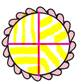(b) How much does each get?

Ans. (b)Each gets 1/4  of the cake.

(c) Out of 4 parts Rajni will get 2 parts. Which is equal to half of the cake. So she can write as 2/4  or 1/2  .

Ans. (c)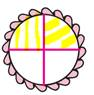(d) Colour the share Raju got.

Ans. (d)5. Using a Price List:(a) How much does 1/2  kg tomato costs?

Ans. (a)Cost of 1 kg tomato = Rs8

Cost of 1/2 kg of tomato = Rs 8/2  = Rs 4

(b) Which costs more 1/2 kg of onions or 1/4  kg of carrots.

Ans. (b)Cost of 1 kg of onions = Rs. 10

Cost of 1/2  kg of onions = Rs. 10/2  = Rs 5

Cost of 1 kg of carrots = Rs 16

Cost of 1/4 kg of carrots = Rs. 16/4 = Rs 4

Since, 5 is more than 4, therefore, cost of 1/2 kg of onions is more than the cost of 1/4 kg of onions.

(c) What is the price of 3/4 kg potato?

Ans. (c)Cost of 1 kg potato = Rs. 12

Cost of 3/4 kg of potato = Rs 12x 3/4

= Rs.  (12x3)/4

= Rs. 9

(d) Keerthi is going for shopping. She has only Rs 20 with her. Can she buy all the things in her shopping list?

Ans. (d)Cost of 1/2 kg potato = Rs. 8 x 1/2 = Rs. 4

Cost of 2 kg pumpkin = Rs. 4 2 = Rs. 8

Cost of 1/4  kg carrot = Rs. 16 x 1/4 = Rs. 4

Total cost = Rs. 16

Since, Keerthi has Rs. 20 which are more than Rs. 16, so she can buy all the things in her shopping list.

6. (a) What part of the whole is coloured? Write below each shape.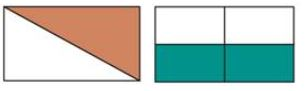Ans. 1/2  is coloured and 2/4  or 1/2  is coloured.

(b) Colour that part of the shapes which is written below.

Ans.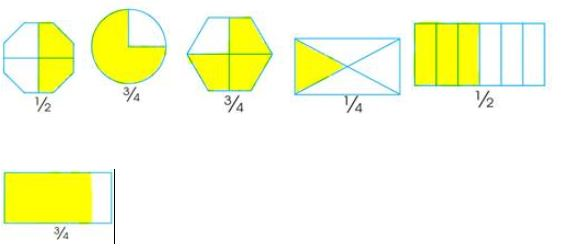7. Match the colour part as shown.

Ans.8. How many centimeters long is the line?

Ans.The line is 50 cm long (because 1 metre = 100 cm)

1 metre = 100 cm

1/2 metre = 50 cm  100 x 1/2 = 50
1/4 metre = 25 cm  100 x 1/4 = 25
3/4 metre = 75 cm  100 x 3/4 = 75

9. (a) How many millimetres of milk does each bottle have?

Ans. (a)Each bottle has (100/4) = 250 ml of milk.

(b) Shade the level of milk in each bottle.

Ans. (b)The level of milk is shaded as shown in the adjacent figure.

(c) How many milliliter of milk does each bottle hold?

Ans. (c)Milk in the first bottle = 3/4 X 1000 ml = 750 ml

Milk in the first bottle = 1/4 x 1000 ml = 250 ml

10. Choose from the weights above to make the two pans equal. In how many ways you can do it?

Ans.Two pans can be made equal by putting the weights shown in the empty pan in many ways. Some of them are given below:

(i)1 kg, 500 g, 500 g

(ii)1 kg, 500 g, 300 g, 200 g

(iii)1 kg, 500 g, 250 g, 250 g

(iv)1 kg, 250 g, 250 g, 250 g

(v)1 kg, 500 g, 200 g, 200 g, 100 g

(vi)1 kg, 500 g, 250 g, 200 g, 50 g

(vii)500 g, 500 g, 250 g, 250 g, 250 g, 200 g, 50 g

(viii)500 g, 500 g, 250 g, 250 g, 200 g, 200 g, 100 g

11.(a) Draw the weights in empty pan. Remember 1 kg = 1000g.

Ans. (a)To balance the two pans, we will put one weight of 1 kg and two weights 500 g in the empty pan

(b) In how many different ways can you balance this weight of 3/4 kg?

Ans. (b)Some of weights which can balance 3/4 kg of weight are as under:

(1) 500 g, 250 g; (2) 250 g, 250 g, 50 g; (3) 250 g, 200 g, 200 g, 100 g.

12. Kannan shaded some parts as shown. But his friend Mini says that it wrong. Explain why it is wrong.

Ans.In the case of the rectangle, the four divisions are not of the equal size. So, cannot be said the shaded part is 1/2 . In case of the triangle, the line is not at the middle.

Clearly, the shaded part is not 1/2 .

13. There are 60 mangoes. 1/2 of them are ripe. How many mangoes are ripe?

Ans.Number of mangoes = 60/2  = 30.

14. There are 32 children. 1/2  of them are girls. How many children are boys?

Ans.Total number of children = 32

Number of girls = 32/2  = 16

Thus, number of boys = 32- 16= 16.

15. There are 20 stars. A quarter of them are red. How many stars are red. How many are not red?

Ans.Total number of stars = 20

Number of red stars = A quarter of 20 stars

=1/4x 20 = 5 stars

Number of non-red stars = 20 – 5 = 15.

16. Ravi wants a pencil. It costs Rs. 2. He gives a one-rupee coin, one half rupee coin and one-quarter rupee coin. Is it enough?

Ans.Cost of a pencil = Rs. 2

Money paid = Re 1.00 + Re 0.50 + Re 0.25 = Rs. 1.75

Since, Rs 2 is more than Rs 1.75, therefore, money paid is not enough.

## Tags:

Click for more Mathematics Study Material

## Latest NCERT & CBSE News

Read the latest news and announcements from NCERT and CBSE below. Important updates relating to your studies which will help you to keep yourself updated with latest happenings in school level education. Keep yourself updated with all latest news and also read articles from teachers which will help you to improve your studies, increase motivation level and promote faster learning

### CBSE Decision on Board Exam 2020

The Class 10 and 12 exams are cancelled, and results for both 10th and 12th would be declared by July 15 based on internal exams. CBSE Class 12 students would have the option to appear for the exams at a later date. CBSE has a scheme in which marks scored in past 3...

### Cogito and The Question Book A series on Thinking skills by CBSE

The Board has dedicated this academic session 2020-21 for ‘Competency Based Learning’. Skills connected to Critical & Creative thinking, Problem Solving, Collaboration and Communication are core to successful living in the 21st Century. To focus on the importance...

### CBSE Reduced Syllabus Clarification

CBSE board has issued the following clarification relating to various news reports regarding reduction in syllabus and questions from the reduced syllabus during board exams. Please read below: It has come to notice of the Board through a section of media reports that...

### Board Exams helpline by CBSE

According to the recent announcement of CBSE, the pending Class 10 and 12 Board exams are going to be held from 01 July to 15 July 2020 in India. Along with this, it has also been decided to resume the free of cost tele-counseling service for students from 9.30 am to 5...

### CBSE Online Training for Teachers 2020

It is clear that this pandemic has utterly disrupted an education system that many assert was already losing its relevance. Enhancing professional development digitally is a need in the times of COVID-19, where social distancing and remote interactions is the new...

### Procedure for correction in Name and Date of Birth

Procedure for correction in Name and Date of Birth in CBSE records Name Change: Applications regarding changes in name or surname of candidates will be considered provided the changes have been admitted by the Court of law and notified in the Government Gazette before...

×